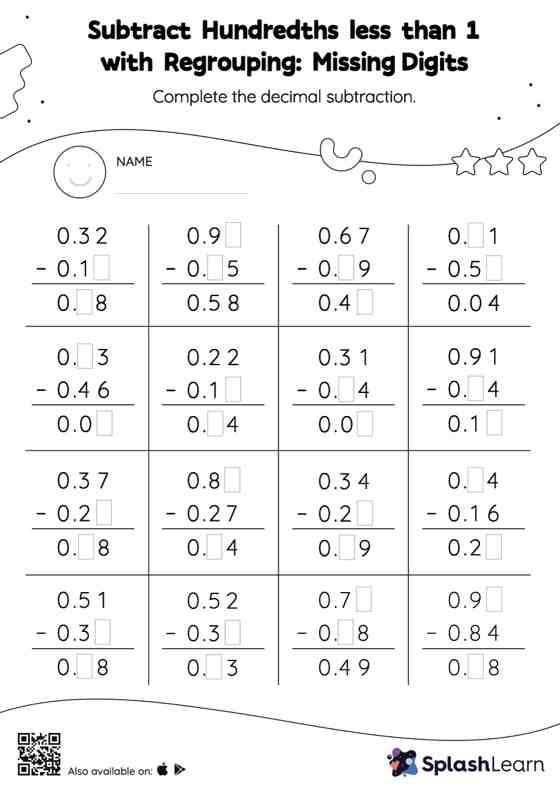# Subtract Hundredths less than 1 with Regrouping: Missing Digits Worksheet

Home > Subtract Hundredths less than 1 with Regrouping: Missing DigitsWhile subtracting decimals, students use the relationship between addition and subtraction to find the missing number. They also need to regroup numbers in subtract hundredths less than 1 with regrouping worksheet utilizing the relationship between ones, tenth, hundredth, thousandth, etc., To get to the result. In each problem, the numbers are laid out in the vertical format. In this format, pair of digits in each successive place are tackled one by one. This helps students follow a structured approach.